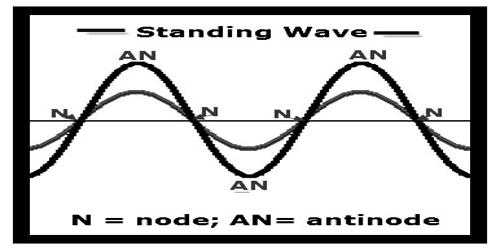Physics

# Characteristics of Stationary WaveCharacteristics of stationary wave:

The resultant wave produced by the superposition of two progressive waves having the same wavelength and amplitude, travelling in opposite direction is called stationary wave. This wave remains confined in that particular region the wave medium and does not propagate through the medium.

There are some characteristics of stationary wave. These are discussed below:

(a) This wave is produced in certain portion of a medium.

(b) Unlike progressive wave it remains confined in a particular place.

(c) Amplitudes at different points of the wave are not the same.

(d) The points where the amplitude is maximum are called antinodes and the points where amplitude is zero are called nodes.

(e) Amplitude of the antinodes a double the amplitude of principal wave.

(f) Displacement of particles between two successive nodes is in the same direction and distance between them is λ/2. Portion between two successive nodes is called a loop.

(g) Displacement between two successive loops is opposite to each other.

(h) In nodes change of pressure and density is maximum, whereas in antinodes change of pressure and density is zero.

(i) Distance between three successive nodes or antinodes or between two loops is called wavelength of a stationary wave.

(j) Except the particles of the nodes all other particles execute the simple harmonic motion.

(k) Wavelength (λ) or frequency (n) of a stationary wave in a medium is equal to the wavelength (λ) or frequency (n) of the principal wave.